Search

Matrix Multiplication / The Identity Matrix (page 3 of 3)Here are a couple more examples of matrix multiplication:

• Find CD and DC, if they exist, given that C and D are the following matrices: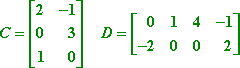C is a 3×2 matrix and D is a 2×4 matrix, so first I'll look at the dimension product for CD: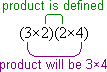So the product CD is defined (that is, I can do the multiplication); also, I can tell that I'm going to get a 3×4 matrix for my answer. Here's the multiplication: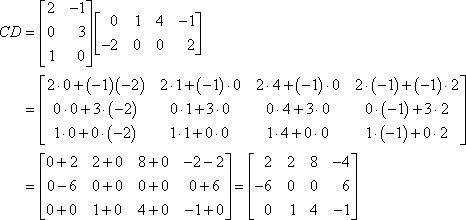However, look at the dimension product for DC: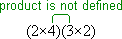Since the inner dimensions don't match, I can't do the multiplication. (The columns of C aren't the same length as the rows of D; the columns of C are too short, or, if you prefer, the rows of D are too long.) Then the answer is: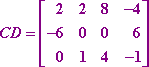DC is not defined.

• Given the following matrices A and B, and defining C as AB = C, find the values of entries c3,2 and c2,3 in matrix C.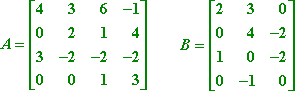The dimension product of AB is (4×4)(4×3), so the multiplication will work, and C will be a 4×3 matrix. But to find c3,2, I don't need to do the whole matrix multiplication. The 3,2-entry is the result of multiplying the third row of A against the second column of B, so I'll just do that:

c3,2 = (3)(3) + (2)(4) + (2)(0) + (2)(1) = 9  8 + 0 + 2 = 3

On the other hand, c2,3 is the result of multiplying the second row of A against the third column of B, so:   Copyright © Elizabeth Stapel 2003-2011 All Rights Reserved

c2,3 = (0)(0) + (2)(2) + (1)(2) + (4)(0) = 0  4  2 + 0 = 6

c3,2 = 3 and c2,3= 6

This type of problem serves as a reminder that, in general, to find ci,j you multiply row i of A against column j of B.

Multiplying by the identity

The "identity" matrix is a square matrix with 1's on the diagonal and zeroes everywhere else. Multiplying a matrix by the identity matrix I (that's the capital letter "eye") doesn't change anything, just like multiplying a number by 1 doesn't change anything. This property (of leaving things unchanged by multiplication) is why I and 1 are each called the "multiplicative identity" (the first for matrix multiplication, the latter for numerical multiplication).

But while there is only one "multiplicative identity" for regular numbers (being the number 1), there are lots of different identity matrices. Why? Because the identity matrix you need for any particular matrix multiplication will depend upon the size of the matrix against which the identity is being multiplied, and perhaps also the side against which you're doing the multiplication (because, for a non-square matrix, right-multiplication and left-multiplication will require a different-size identity matrix).

For instance, suppose you have the following matrix A: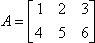To multiply A on the right by the identity (that is, to do AI ), you have to use I3, the 3×3 identity, in order to have the right number of rows for the multiplication to work: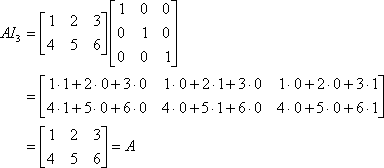On the other hand, to multiply A on the left by the identity, you have to use I2, the 2×2 identity, in order to have the right number of columns: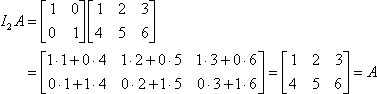That is, if you are dealing with a non-square matrix (such as A in the above example), the identity matrix you use will depend upon the side that you're multiplying on. This is just another example of matrix weirdness. Don't let it scare you. Matrices aren't bad; they're just different... really, really different.

<< Previous  Top  |  1 | 2 | 3  |  Return to Index

 Cite this article as: Stapel, Elizabeth. "Matrix Multiplication / The Identity Matrix." Purplemath. Available from     https://www.purplemath.com/modules/mtrxmult3.htm. Accessed [Date] [Month] 2016

Study Skills Survey

Tutoring from Purplemath
Find a local math tutor